Test: Graph Theory- 1

# Test: Graph Theory- 1 - Electrical Engineering (EE)

Test Description

## 10 Questions MCQ Test GATE Electrical Engineering (EE) 2024 Mock Test Series - Test: Graph Theory- 1

Test: Graph Theory- 1 for Electrical Engineering (EE) 2023 is part of GATE Electrical Engineering (EE) 2024 Mock Test Series preparation. The Test: Graph Theory- 1 questions and answers have been prepared according to the Electrical Engineering (EE) exam syllabus.The Test: Graph Theory- 1 MCQs are made for Electrical Engineering (EE) 2023 Exam. Find important definitions, questions, notes, meanings, examples, exercises, MCQs and online tests for Test: Graph Theory- 1 below.
Solutions of Test: Graph Theory- 1 questions in English are available as part of our GATE Electrical Engineering (EE) 2024 Mock Test Series for Electrical Engineering (EE) & Test: Graph Theory- 1 solutions in Hindi for GATE Electrical Engineering (EE) 2024 Mock Test Series course. Download more important topics, notes, lectures and mock test series for Electrical Engineering (EE) Exam by signing up for free. Attempt Test: Graph Theory- 1 | 10 questions in 30 minutes | Mock test for Electrical Engineering (EE) preparation | Free important questions MCQ to study GATE Electrical Engineering (EE) 2024 Mock Test Series for Electrical Engineering (EE) Exam | Download free PDF with solutions
 1 Crore+ students have signed up on EduRev. Have you?
Test: Graph Theory- 1 - Question 1

### A non-planar graph with minimum number of vertices has

Detailed Solution for Test: Graph Theory- 1 - Question 1

K5 is smallest non-planar graph in terms of number of vertices.
The number of vertices in K5 is 5 and number of edges in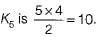Test: Graph Theory- 1 - Question 2

### Maximum number of edges in a n-node undirected graph without self loops is

Detailed Solution for Test: Graph Theory- 1 - Question 2

The graph containing maximum number of edges in a n-node undirected graph without self loops is complete graph.
The number of edges in complete graph with n-node,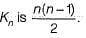Test: Graph Theory- 1 - Question 3

### The minimum number of colours required to colour the following graph, such that no two adjacent vertices are assigned the same colour, is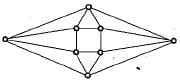Detailed Solution for Test: Graph Theory- 1 - Question 3

An assignment of the colors 1,2,3 and 4 to the vertices of the graph is shown below such that the graph is properly colored.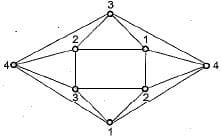So 4 colours are required.
[Note: The graph is a planar graph. The four colour theorem says that the chromatic number of a planar graph is at most = 4]

Test: Graph Theory- 1 - Question 4

What is the maximum number of edges in an acyclic undirected graph with n vertices?

Detailed Solution for Test: Graph Theory- 1 - Question 4

Acyclic graph are graphs which does not contain cycle.
For maximum number of edges each vertex connect to other vertex only if it does not from a cycle i.e. from a tree with n vertices, which has maximum 77-1 edges. Ex. with 4 vertices, 3 edges maximum.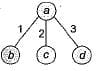Test: Graph Theory- 1 - Question 5

Which one of the following graphs is NOT planner?

Detailed Solution for Test: Graph Theory- 1 - Question 5

G1 is same as K3 3 which is known to be non planar G2, G3 and G4 can be redrawn as follows so that they are planar.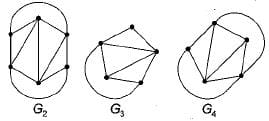Test: Graph Theory- 1 - Question 6

Let G be the non-planar graph with the minimum possible number of edges. Then G has

Detailed Solution for Test: Graph Theory- 1 - Question 6

K5 and K3, 3 are the smallest non planar graphs. K5 has 5 vertices and 5C2 = 10 edges and K3, 3 has 6 vertices and 3 x 3 = 9 edges . So, the non planar graph with minimum number of edges is K3, 3 with 9 edges and 6 vertices.
[Note: K5 is the non planar graph with minimum number of vertices]

Test: Graph Theory- 1 - Question 7

Which one of the following is TRUE for any simple connected undirected graph with more than 2 vertices?

Detailed Solution for Test: Graph Theory- 1 - Question 7

In a simple connected undirected graph (with more than two vertices), at least 2 vertices must have same degree, since if this is not true, then all vertices would have different degrees, A graph with all vertices having different degrees is not possible to construct (can be proved as a corollary to the Havell-Hakimi theorem). Notice that it is possible to construct graphs satisfying choices a, c and d.

Test: Graph Theory- 1 - Question 8

In a binary tree with n nodes, every node has an odd number of descendants. Every node is considered to be its own descendant. What is the number of nodes in the tree that have exactly one child?

Detailed Solution for Test: Graph Theory- 1 - Question 8

A tree with 1 node is not possible, since it is given that every node has exactly 1 child.
Now consider a tree with 2 nodes (a is the root)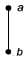Now a has exactly one child. Number of descendents of a = 2. But this contradicts the given fact that every node has an odd number of descendents.
Now consider atree with 3 nodes. Since, every- node has exactly one child, it must be of the form,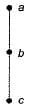Here a has 3 descendents, b has 2 descendents and c has one. Again we have contradiction in that b does not have odd number of descendents. Similarly can show that for tree with 4, 5, 6 ... nodes, it is not possible to have all nodes with odd number of descendents! So correct answer is the tree has 0 nodes, i.e., choice (a).

Test: Graph Theory- 1 - Question 9

The degree sequence of a simple graph is the sequence of the degrees of the nodes in the graph in decreasing order. Which of the following sequences can not be the degree sequence of any graph?​
I. 7, 6, 5, 4, 4, 3, 2, 1
lI. 6, 6,6, 6, 3, 3, 2, 2
III. 7, 6, 6, 4, 4, 3, 2, 2
IV. 8, 7, 7, 6, 4,2, 1,1

Detailed Solution for Test: Graph Theory- 1 - Question 9

Havell-Hakimi algorithm can be used to check whether a given degree sequence is a graph or not.
The algorithm is
1. Remove top node of the sequence.
2. Subtract "1" from as m any nodes in remaining sequence as the degree of top node that was removed.
3. Rearrange this sequence in non increasing order.
4. Check if resulting sequence is a graph.
5. Proceed again to step 1.
If the given sequence is not a graph we will see a violation in step 4, such as presence of negative degrees in the sequence. Otherwise the algorithm will bottom out with a degree sequence consisting of only even number of 1 ’s and any number of 0’s.
Now applying the algorithm to the degree sequences I, II, III and IV, one by one: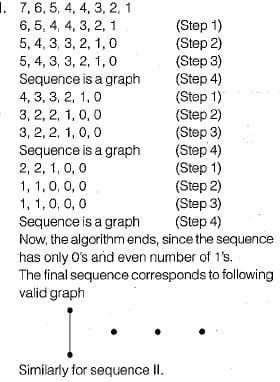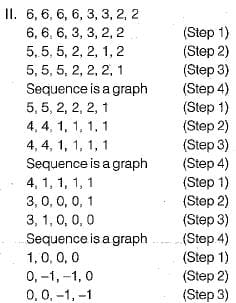The sequence is not a graph (Step 4), since negative degrees not possible in a valid graph. So, algorithm ends.
II is cannot be the degree sequence of any graph. Similarly we can show that III is degree sequence of some graph and IV is not a degree sequence of any graph.

Test: Graph Theory- 1 - Question 10

K4 and Q3 are graphs with the following structure: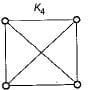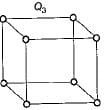Which one of the following statements is TRUE in relation to these graphs?

Detailed Solution for Test: Graph Theory- 1 - Question 10

The planar embedding of K4 and Q3 is shown below: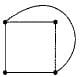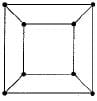So both graphs are planar.

## GATE Electrical Engineering (EE) 2024 Mock Test Series

23 docs|285 tests
Information about Test: Graph Theory- 1 Page
In this test you can find the Exam questions for Test: Graph Theory- 1 solved & explained in the simplest way possible. Besides giving Questions and answers for Test: Graph Theory- 1, EduRev gives you an ample number of Online tests for practice

## GATE Electrical Engineering (EE) 2024 Mock Test Series

23 docs|285 tests